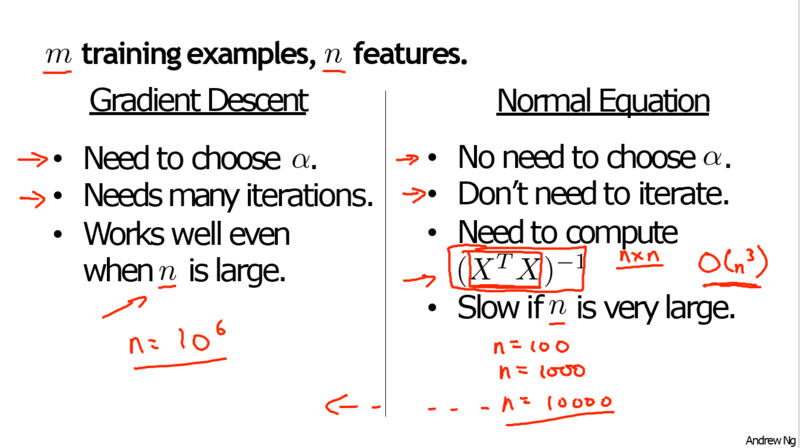X = \begin{bmatrix} 1 && 2104 && 5 && 1 && 45 \
1 && 1416 && 3 && 2 && 40 \
1 && 1534 && 3 && 2 && 30 \
1 && 852 && 2 && 1 && 36 \end{bmatrix}

Y = \begin{bmatrix} 460 \
232 \
315 \
178
\end{bmatrix}

\theta = (X^TX)^{-1} X^Ty

x^(i) = \begin{bmatrix}
x_0^(i)\
x_1^(i)\
x_2^(i)\
…\
x_n^(i)\
\end{bmatrix}

(n+1) 维矩阵：
X = \begin{bmatrix}
… && (x^(1))^T && …\
… && (x^(2))^T && …\
… && (x^(3))^T && …\
…\
… && (x^(m))^T && …\
\end{bmatrix}

x^(i) = \begin{bmatrix}
1 \
x^(i)
\end{bmatrix}

X = \begin{bmatrix}
1 && x_1^(1)\
1 && x_2^(1) \
… \
1 && x_m^(1)
\end{bmatrix}

y = \begin{bmatrix}
y^(1) \
y^(2) \
… \
y^(m)
\end{bmatrix}

\theta = (X^TX)^{-1} X^Ty（懒得手写了就直接赋值吴恩达老师的图了），这里就提一下两者的最后一个特点。由于矩阵X是m*(n+1)维的矩阵，当我们的特征值非常多的时候，计算(X^TX)^{-1}时候的计算量是非常庞大的，因此计算的速度会非常慢，但n的大小很显然跟梯度下降的速度没关系，所以梯度下降算法依然可以正常运行。这里吴恩达老师是建议在n=10000的时候就考虑一下是否要使用梯度下降法了，10000以下都可以使用正规方程，当然这里还是需要通过实际情况来决定。